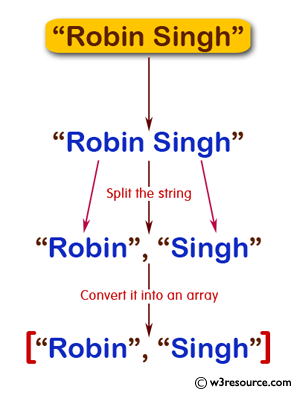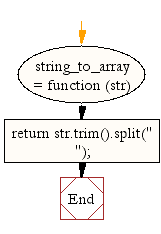# JavaScript: Split a string and convert it into an array of words

## JavaScript String: Exercise-3 with Solution

Write a JavaScript function to split a string and convert it into an array of words.

Test Data :
console.log(string_to_array("Robin Singh"));
["Robin", "Singh"]

Pictorial Presentation:Sample Solution:-

HTML Code:

``````<!DOCTYPE html>
<html>
<head>
<meta charset="utf-8">
<title>Split a string and convert it into an array of words.</title>
</head>
<body>
</body>
</html>
```
```

JavaScript Code:

``````string_to_array = function (str) {
return str.trim().split(" ");
};
console.log(string_to_array("Robin Singh"));
```
```

Sample Output:

```["Robin","Singh"]
```

Flowchart:Live Demo:

See the Pen JavaScript Split a string and convert it into an array of words - string-ex-3 by w3resource (@w3resource) on CodePen.

Contribute your code and comments through Disqus.

What is the difficulty level of this exercise?

﻿

## JavaScript: Tips of the Day

Returns the number of times a function executed per second. hz is the unit for hertz, the unit of frequency defined as one cycle per second

Example:

```const tips_hz = (fn, iterations = 100) => {
const before = performance.now();
for (let i = 0; i < iterations; i++) fn();
return (1000 * iterations) / (performance.now() - before);
};
// 10,000 element array
const numbers = Array(10000)
.fill()
.map((_, i) => i);

// Test functions with the same goal: sum up the elements in the array
const sumReduce = () => numbers.reduce((acc, n) => acc + n, 0);
const sumForLoop = () => {
let sum = 0;
for (let i = 0; i < numbers.length; i++) sum += numbers[i];
return sum;
};

// 'sumForLoop' is nearly 10 times faster
console.log(Math.round(tips_hz(sumReduce)));
console.log(Math.round(tips_hz(sumForLoop)));
```

Output:

```4004
990
```